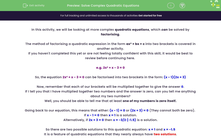### Comprehensive & curriculum aligned

In this worksheet, students will learn how to solve a quadratic equation of the form ax² + bx + c = 0 by factorising (including equations which require rearranging).Key stage:  KS 4

Year:  GCSE

GCSE Subjects:   Maths

GCSE Boards:   Pearson Edexcel, OCR, Eduqas, AQA,

Curriculum topic:   Algebra

Curriculum subtopic:   Solving Equations and Inequalities Algebraic Equations

Popular topics:   Factors worksheets

Difficulty level:#### Worksheet Overview

In this activity, we will be looking at more complex quadratic equations, which can be solved by factorising.

The method of factorising a quadratic expression in the form ax² + bx + c into two brackets is covered in another activity.

If you haven't completed this yet or are not feeling totally confident with this skill, it would be best to review before continuing here.

e.g. 2x² + x - 3 = 0

So, the equation 2x² + x - 3 = 0 can be factorised into two brackets in the form: (x - 1)(2x + 3)

Now, remember that each of our brackets will be multiplied together to give the answer 0.

If I tell you that I have multiplied together two numbers and the answer is zero, can you tell me anything about my two numbers?

Well, you should be able to tell me that at least one of my numbers is zero itself.

Going back to our equation, this means that either: (x - 1) = 0 or (2x + 3) = 0 ​(They cannot both be zero).

If x - 1 = 0 then x = 1 is a solution.

Alternatively, if 2x + 3 = 0 then x = -3/2 (-1.5) is a solution.

So there are two possible solutions to this quadratic equation: x = 1 and x = -1.5

It is a feature of quadratic equations that they nearly always have two solutions.

Here's another example, this time we'll just include the lines of working you would need to show:

6n² - 5n - 4 = 0

(2n + 1)(3n - 4) = 0

Either 2n + 1 = 0 or 3n - 4 = 0

So n = -1/2 or n = 4/3

Finally, we'll look at one more example:

4z(z - 1) = 3

Now, this equation looks like it has already been factorised.

However, you will notice the right-hand side is 3, not 0.

Quadratic equations can only be solved if the right-hand side is zero.

So we need to rearrange the equation first and this means we must expand the brackets as our starting point:

4z² - 4z = 3

Now subtract 3 from both sides:

4z² - 4z - 3 = 0

Now our equation is in the correct form for us to factorise into two brackets and then solve:

4z² - 4z - 3 = 0

(2z - 3)(2z + 1) = 0

Either 2z - 3 = 0 or 2z + 1 = 0

So z = 3/2 or z = -1/2

Ready to try some for yourself now?

In this activity, we will solve quadratic equations by ensuring that they conform to the style of ax² + bx + c = 0 first. Then, factorising them to find possible values for our variables.

### What is EdPlace?

We're your National Curriculum aligned online education content provider helping each child succeed in English, maths and science from year 1 to GCSE. With an EdPlace account you’ll be able to track and measure progress, helping each child achieve their best. We build confidence and attainment by personalising each child’s learning at a level that suits them.

Get started